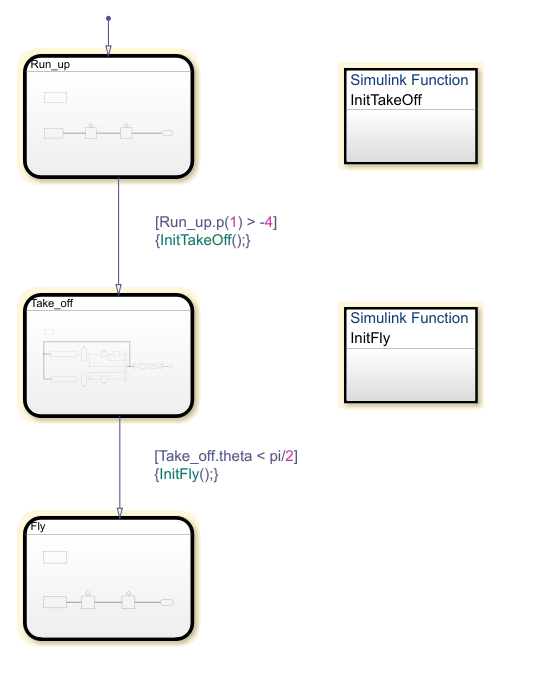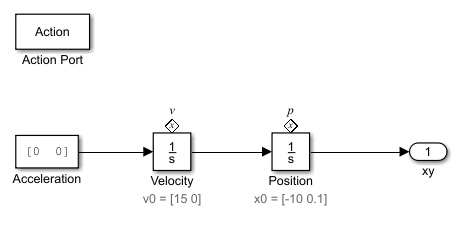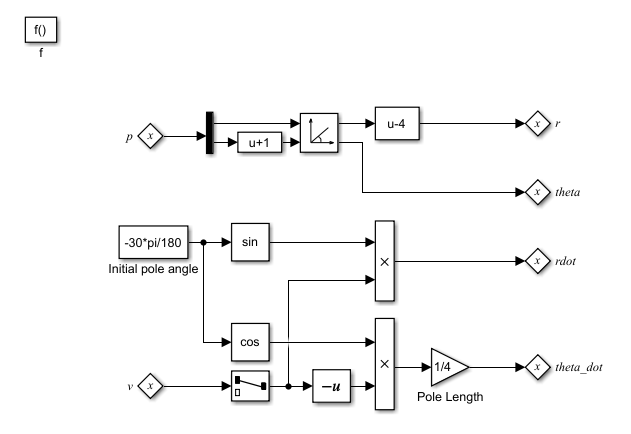Documentation

## Simulink Subsystems as States

By using a Simulink® subsystem within a Stateflow® state, you can model hybrid dynamic systems or systems that switch between periodic or continuous time dynamics. In your Stateflow chart, you can use Simulink based states to model a periodic or continuous dynamic system combined with switching logic that uses transitions. You can access inputs and outputs from your chart within each Simulink based state. Simulink based states are supported only in Stateflow charts in a Simulink model.

To initialize Simulink blocks when switching between Simulink based states, use Stateflow textual notation or Simulink State Reader and State Writer blocks.

To create linked Simulink based states, use libraries to save action subsystems. When you copy an action subsystem from a library model into a Stateflow chart, it appears as a linked Simulink based state. When you update the library block, the changes are reflected in all Stateflow charts containing the block.

Using Simulink based states means that you do not have to use complex textual syntax in Stateflow to model hybrid systems.

### When to Use Simulink Based States

Use Simulink based states when:

• You want to model hybrid dynamic systems that include continuous or periodic dynamics.

• The structure of the system dynamics change substantially between the various modes of operation, for example, modeling PID controllers.

For systems where you call logic intermittently, use Simulink functions.

When the structure of the Simulink algorithm remains substantially unchanged, but certain gains or parameters switch between various models, use Simulink logic outside of Stateflow. An example of this type of algorithm is gain scheduling. See Model Gain-Scheduled Control Systems in Simulink (Simulink Control Design).

### Model a Pole Vaulter by Using Simulink Based States

This Stateflow chart models a person moving through the stages of pole vaulting by using Simulink based states. The first stage is the approach run of the vaulter, which is modeled in the Simulink based state `Run_up`. In the second stage, the vaulter plants the pole and takes off, which is modeled by the Simulink based state `Take_off`. The final stage happens when the vaulter clears the bar and releases the pole, which is modeled by the Simulink based state `Fly`.The Stateflow chart contains this logic:The states `Run_up` and `Fly` are easier to model by using Cartesian coordinates. The state `Take_off` is easier to model by using polar coordinates. To switch from one coordinate system to another, use Simulink functions `InitTakeOff` and `InitFly`.

#### Model the Approach of the Pole Vaulter

The Simulink based state `Run_up` contains this logic:The default state in the chart `PoleVaulter` is `Run_up`. This state models the pole vaulter traveling along the ground toward the jump. The pole vaulter starts at -10 on the x-axis and runs toward zero. As the pole vaulter moves along the ground, the position of the pole vaulter in the xy-plane is continuously changing, but the state of running remains the same. In this model, the integrator blocks `Position` and `Velocity` are state owner blocks for State Reader blocks in the Simulink function `InitTakeOff`. This subsystem outputs the Cartesian coordinates of the pole vaulter.

#### Convert Cartesian Coordinates to Polar Coordinates

The Simulink function `InitTakeOff` contains this logic:Once the position of the pole vaulter along the x-axis, `Run_up.p(1)`, becomes greater than `-4`, the transition from `Run_up` to `Take_off` occurs. During the transition `InitTakeOff` is initialized, the State Reader block connects to its owner block, and the function is executed. This function converts the Cartesian coordinates from `Position` and `Velocity` to polar coordinates, `r`, `theta`, `rdot`, and `theta_dot`. These coordinates are output as State Writer blocks, which are connected to owner blocks in the state `Take_off`.

#### Model the Take Off of the Pole Vaulter

The Simulink based state `Take_off` contains this logic:Once the position of the pole vaulter along the x-axis, `Run_up.p(1)`, becomes greater than `-4`, the active state becomes `Take_off`. This Simulink subsystem models the pole vaulter during the take off phase of the jump. The subsystem outputs the Cartesian coordinates of the pole vaulter.

#### Convert Polar Coordinates to Cartesian Coordinates

The Simulink function `InitFly` contains this logic:Once the angle of the pole vaulter, `theta`, becomes less than `pi/2`, the transition from `Take_off` to `Fly` occurs. During the transition `InitFly` is initialized, the State Reader block connects to its owner block, and the function is executed. This function converts the polar coordinates from `r`, `theta`, and `theta_dot` to Cartesian coordinates, `xy_integ` and `xydot`. These coordinates are output as State Writer blocks, which are connected to owner blocks in the state `Fly`.

#### Model the Free Fall of the Pole Vaulter

The Simulink based state `Fly` contains this logic:Once the angle of the pole vaulter, `theta`, is less than `pi/2`, the active state becomes `Fly`. This state models the pole vaulter after the jump has cleared and the pole vaulter is falling to the ground. As the pole vaulter falls, the position of the pole vaulter in the x-y plane is continuously changing, but the state of falling remains the same. In this model, the integrator blocks `xydot` and `xy_integ` are state owner blocks for State Writer blocks in the Simulink function `InitFly`. This subsystem outputs the Cartesian coordinates of the pole vaulter.

The results of this simulation are seen in the XY Graph.### Limitations

You cannot use Simulink based states with:

• Moore charts

• Discrete Event charts

• HDL Coder

• PLC Coder

• Simulink Code Inspector

• Super step transitions

Simulink based states do not support debugging.

## Related Topics

##### SupportGet trial now